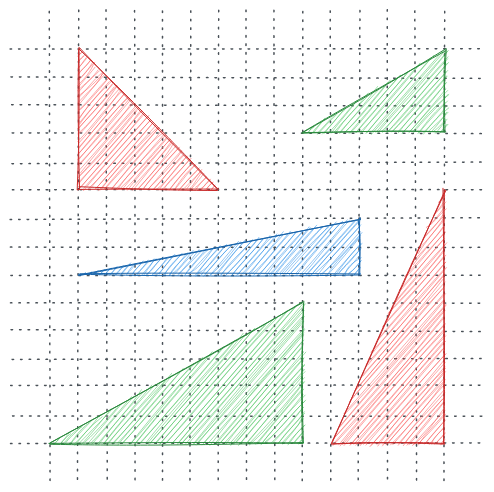# Game of TriangleszobayerRMSTU Bangabandhu Online...
Limits 1s, 512 MB

In order to overcome the boredom during the Covid19 lockdown, you have created a small but clever game, very aptly named, “The Game of Triangles”. The idea is not too complicated. Your program randomly generates a lot of triangles. Each of these triangles is represented in the Cartesian coordinate system with vertices in integer coordinates. But the game hides the triangle from the players. Players take turns and cut the coordinate system (or the 2D plane) with a straight line along either the x-axis or the y-axis. The game continues for as many rounds as you want. In each round, you calculate your score by calculating the number of triangles affected by your cut. A triangle is affected by a cut if both the right side and the left side areas of the cut have positive areas.However, you quickly realize that this is a very difficult task, especially when your game starts producing a lot of triangles. So now you need to write a program to calculate the number of affected triangles for a given cut.

## Input

The first line of the input is the number of test cases $T$ ($T \le 20$).

Each test case starts with a positive integer $N$ ($2 \le N \le 10^5$), the number of generated triangles.

The following 6 integers represent the three coordinates of the triangle $(x1, y1)$, $(x2, y2)$, $(x3, y3)$ in no particular order. It is guaranteed that the triangles will have a positive area, but they can overlap one another arbitrarily.

The following line contains a positive integer $M$ ($2 \le M \le 10^5$), the number of cuts. Each of the following M lines contains a cut in the form of $s$, $v$ where s is either ‘x’ or ‘y’ denoting the axis of alignment, and $v$ is the coordinate value. This represents either the $x = v$ or $y = v$ line equation based on the value of s.

It is guaranteed that $0 <= x1, y1, x2, y2, x3, y3, v < 10^6$.

## Output

For each test case, first print the test case serial number on a line, followed by $M$ lines, each containing an integer denoting the number of affected triangles for the corresponding cut in the input. Please check the sample input-output section for formatting details.

## Sample

InputOutput
2
3
1 0 0 2 2 2
1 3 3 5 4 0
5 4 4 5 4 4
4
x 4
x 1
y 3
y 1
4
2 7 6 0 0 5
7 1 7 10 11 11
5 10 2 9 6 8
1 9 10 10 4 1
4
y 6
x 2
x 4
x 9

Case 1:
0
1
1
2
Case 2:
3
2
3
2


The input file is very large, please use faster IO.

### Submit